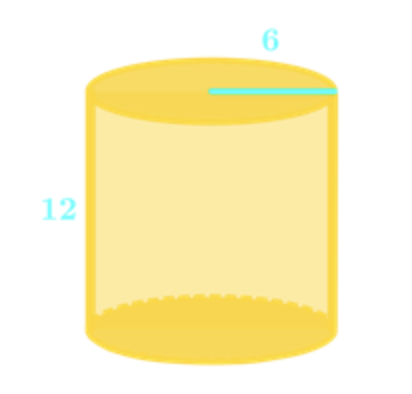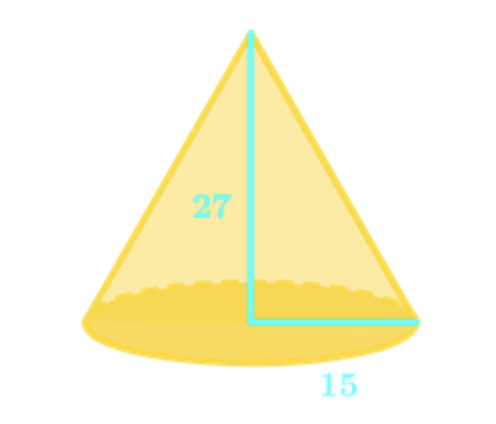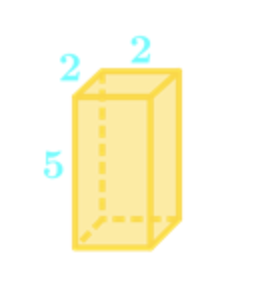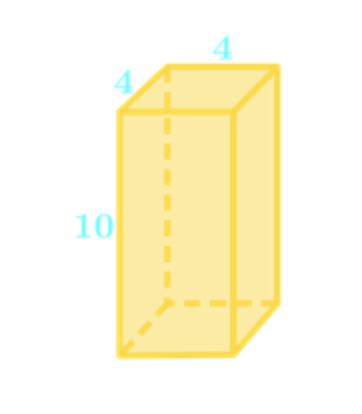# Similar solids#### Everything You Need in One Place

Homework problems? Exam preparation? Trying to grasp a concept or just brushing up the basics? Our extensive help & practice library have got you covered.#### Learn and Practice With Ease

Our proven video lessons ease you through problems quickly, and you get tonnes of friendly practice on questions that trip students up on tests and finals.#### Instant and Unlimited Help

Our personalized learning platform enables you to instantly find the exact walkthrough to your specific type of question. Activate unlimited help now!

##### Intros
###### Lessons
1. Introduction to Similar Solids
2. When are two objects similar?
3. Scale factor vs. surface area ratio vs. volume ratio
##### Examples
###### Lessons
1. Identify Similar Solids

Which of the following are similar solids?

1.2.2. Proof of the Relationships Between Scale Factor, Area Ratio and Volume Ratio

Use the following similar solids to prove the relationships between the scale factor, surface area ratio and volume ratio.1. Given the Scale Factors, Find a Surface Area

Given two similar hemispheres. The radius of the smaller hemisphere is $5m$ and that of the larger hemisphere is $7m$. If the surface area of the larger hemisphere is $147m^{2}$, what is the surface area of the smaller hemisphere?

1. Given the Volumes, Find the Scale Factors

Given that the volumes of the two similar prisms are $729cm^{3}$ and $1331cm^{3}$ respectively. What is the scale factor of the smaller prism to the larger prism?

1. Scale Factors Doubled, Find a Volume

The dimensions of a pyramid figure with a volume of $24m^{3}$ have been doubled. What is the volume of the new pyramid figure?

###### Topic Notes

In this lesson, we will learn:

• Identify Similar Solids
• Proof of the Relationships Between Scale Factor, Area Ratio and Volume Ratio
• Given the Scale Factors, Find a Surface Area
• Given the Volumes, Find the Scale Factors
• Scale Factors Doubled, Find a Volume

• Solid: A three-dimensional object
• Two solids are similar when the ratios of their corresponding measures are constant.
• Scale factor:
• The ratios of the corresponding measures of two objects.
• A numeric multiplier used for scaling.
• If two similar solids have a scale factor of $\frac{a}{b}$, then
1. They have a surface area ratio of $(\frac{a}{b})^{2}$.
2. They have a volume ratio of $(\frac{a}{b})^{3}$.